# A disabled automobile is pulled by means of two ropes as shown. The tension in rope AB is 2.2 kN. and the angle alpha is 25 degree. Knowing that the resultant of the two forces applied at A is directed along the axis of the automobile, determine by trigonometry (a) the tension in rope AC, (b) the magnitude of the resultant of the two forces applied at A.

Question-AnswerCategory: Engineering MechanicsA disabled automobile is pulled by means of two ropes as shown. The tension in rope AB is 2.2 kN. and the angle alpha is 25 degree. Knowing that the resultant of the two forces applied at A is directed along the axis of the automobile, determine by trigonometry (a) the tension in rope AC, (b) the magnitude of the resultant of the two forces applied at A.

### A disabled automobile is pulled by means of two ropes as shown. The tension in rope AB is 2.2 kN. and the angle alpha is 25 degree. Knowing that the resultant of the two forces applied at A is directed along the axis of the automobile, determine by trigonometry (a) the tension in rope AC, (b) the magnitude of the resultant of the two forces applied at A.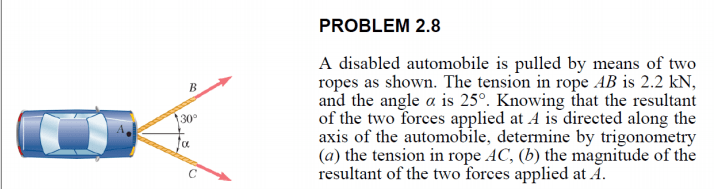Step: 1

Draw the schematic figure.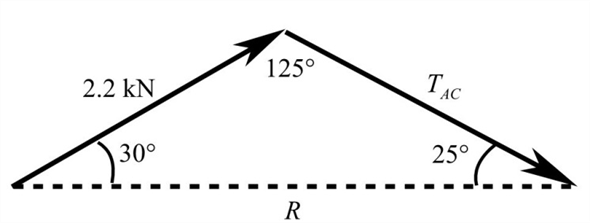Step: 2

Apply sine rule.Here, R is the resultant and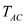is the tension in rope AC.
Calculate the tension in rope AC using the equation.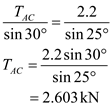Therefore, the tension in rope AC is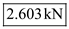.

Step: 3

Calculate the magnitude of the resultant force using the equation.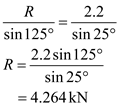Therefore, the magnitude of the resultant force is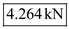.